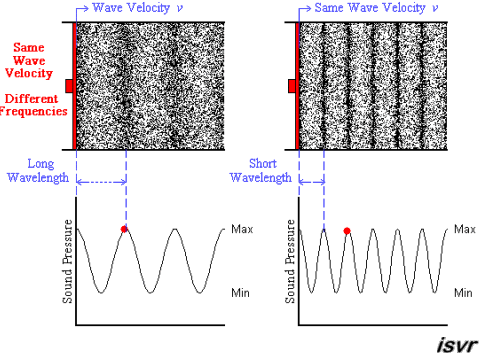# Question f2711

Feb 2, 2014

The wavelength decreases as the frequency increases.

#### Explanation:

The wavelength λ is the distance between successive peaks in a wave.

The frequency $f$ of a wave is the number of complete waves that pass a point in a given time.Above we see two sound waves travelling through space at the same velocity $v$.

The red dot follows the amplitude of the wave as it goes by.

The frequency of oscillation of the dot is the frequency $f$ of the wave. The second wave has twice the frequency of the first.

You can see that the wavelength is halved when the frequency is doubled.

The frequency $f$ and wavelength λ are related to one another and to the speed of the wave $v$ by the formula

fλ = v.

Since $v$ is constant, if $f$ increases, λ# must decrease, and vice versa.